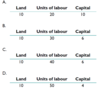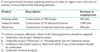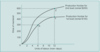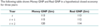Practice Final Examination 1 Flashcards Preview

Economics > Practice Final Examination 1 > Flashcards

Flashcards in Practice Final Examination 1 Deck (30):
1

2

3

4

The table below shows how the factors of production may be combined to produce 100 units of a certain good. Using only the information in the table, which one of the methods can be dismissed by the producer on the grounds of technical inefficiency?5

6

A consumer’s typical demand curve for a good is downward-sloping to the quantity axis because at higher prices the consumerI. encounters a leftward-shifting supply curve for that good, resulting in a lesser equilibrium quantity.II. buys less of the good in place of other goods, which are now relatively less expen-sive.Which of the following is correct?A. I only. B. II only. C. Both I and II. D. Neither I nor II.

The correct answer is B. A demand curve shows the quantity of a good that a consumer would buy at different prices, cet. par. This is independent of the condi- tions of supply; therefore statement I is incorrect. As the price increases, the quantity typically decreases for a number of reasons, one of which is that other goods become relatively more attractive; this is the substitution effect. Thus statement II is correct.

78

9

The diagram shows two production frontiers for catching mackerel, in other words the maximum amount of fish that can be caught with two different amounts of a fixed factor of production (boat) and with different amounts of a variable factor of production (labour). The rental costs of the boats are \$100 and \$200 respectively. All units of labour are identical.I. A movement from X to Y exhibits increasing marginal returns to labour.II. A movement from X to Z exhibits increasing returns to scale.Which of the following is correct?A. I only. B. II only. C. Both I and II. D. Neither I nor II.10

11

12

13

14

15

16

17

18

The following table shows Money GNP and Real GNP in a hypothetical closed economy for three years:Assuming a constant number of workers, it follows that I. the supply of money increased by \$7 million between Year t and Year t + 2. II. output per worker was higher in Year t + 2 than in Year t. Which of the following is correct?A. I only. B. II only. C. Both I and II. D. Neither I nor II.19

20

21

22

23

24

25

26

27

28

29

30